# Probabilities

If probabilities of A, B and A ∩ B are P (A) = 0.62 P (B) = 0.78 and P (A ∩ B) = 0.26 calculate the following probability (of union. intersect and opposite and its combinations):

Correct result:

P(A′) =  0.38
P(B′) =  0.22
P(A ∪ B) =  1.14
P(A′∩ B) =  0.52
P(A ∩ B′) =  0.36
P[( A ∪ B)′] =  -0.14
P( A′ ∪ B) =  0.64

#### Solution:

P(A′) = 1-0.62 = 0.38
P(B′) = 1-0.78 = 0.22
P(A ∪ B) = 0.62+0.78-0.26 = 1.14
P(A′∩ B) = 0.78-0.26 = 0.52
P(A ∩ B′) = 0.62-0.26 = 0.36
P[( A ∪ B)′] = 1-(0.62+0.78-0.26) = -0.14
P( A′ ∪ B) = 1-0.62+ 0.26 = 0.64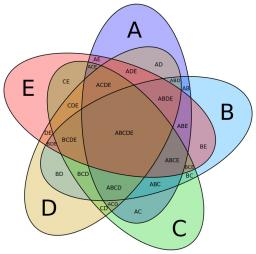We would be pleased if you find an error in the word problem, spelling mistakes, or inaccuracies and send it to us. Thank you!Tips to related online calculators
Would you like to compute count of combinations?

## Next similar math problems:

• Median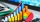The number of missed hours was recorded in 11 pupils: 5,12,6,8,10,7,5,110,2,5,6. Determine the median.
• Three unknownsSolve the system of linear equations with three unknowns: A + B + C = 14 B - A - C = 4 2A - B + C = 0
• ExaminationThe class is 21 students. How many ways can choose two to examination?
• SequenceWrite the first 6 members of these sequence: a1 = 5 a2 = 7 an+2 = an+1 +2 an
• Guppies for sale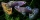Paul had a bowl of guppies for sale. Four customers were milling around the store. 1. Rod told paul - I'll take half the guppies in the bowl, plus had a guppy. 2. Heather said - I'll take half of what you have, plus half a guppy. The third customer, Nancy
• ChordsHow many 4-tones chords (chord = at the same time sounding different tones) is possible to play within 7 tones?
• CardsThe player gets 8 cards of 32. What is the probability that it gets a) all 4 aces b) at least 1 ace
• AP - simpleDetermine the first nine elements of sequence if a10 = -1 and d = 4
• Lottery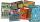Fernando has two lottery tickets each from other lottery. In the first is 973 000 lottery tickets from them wins 687 000, the second has 1425 000 lottery tickets from them wins 1425 000 tickets. What is the probability that at least one Fernando's ticket
• Hearts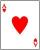5 cards are chosen from a standard deck of 52 playing cards (13 hearts) with replacement. What is the probability of choosing 5 hearts in a row?
• CandiesIn the box are 12 candies that look the same. Three of them are filled with nougat, five by nuts, four by cream. At least how many candies must Ivan choose to satisfy itself that the selection of two with the same filling? ?
• BallsThe urn is 8 white and 6 black balls. We pull 4 randomly balls. What is the probability that among them will be two white?
• First manWhat is the likelihood of a random event where are five men and seven women first will leave the man?
• What is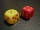What is the probability that the sum of 9 will fall on a roll of two dice? Hint: write down all the pairs that can occur as follows: 11 12 13 14 15. . 21 22 23 24. .. . 31 32. .. . . . . . .. . 66, count them, it's the variable n variable m: 36, 63,. .. .
• Class - boys and girls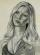In the class are 60% boys and 40% girls. Long hair has 10% boys and 80% girls. a) What is the probability that a randomly chosen person has long hair? b) The selected person has long hair. What is the probability that it is a girl?
• Probability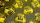What are the chances that the lottery, in which the numbers are drawn 5 of 50 you win the first prize?
• Today in schoolThere are 9 girls and 11 boys in the class today. What is the probability that Suzan will go to the board today?